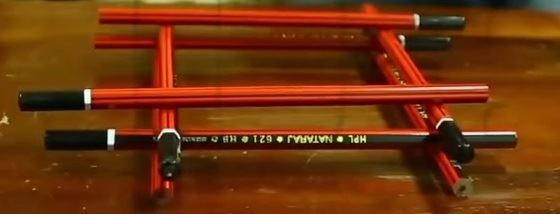Checkout JEE MAINS 2022 Question Paper Analysis : Checkout JEE MAINS 2022 Question Paper Analysis :

# Algebra As Patterns

If you think of Mathematics as a language, then Algebra would be that part of the language which describes various patterns around us. If there is any repetitive pattern, then we can use algebra to simplify this and have a general expression to describe this pattern. Algebraic thinking starts as soon as pupils notice the regular change and attempt to define it. Suppose we can represent algebraic thinking by everyday situations such as balancing concrete elements using balance bins. This kind of activity encourages using more symbolic illustrations in the higher levels when we use letters to generalize thinking or deliberate situations with the help of variables.

## Algebra and Patterns

To understand the relationship between patterns and algebra, we need to try making some patterns. We can use pencils to construct a simple pattern and understand how to create a general expression to describe the entire pattern. It would be best if you had a lot of pencils for this. It will help if they are of similar height.

Find a solid surface and arrange two pencils parallel to each other with some space in between them. Add a second layer on top of it and another on top of that, as shown in the image given below.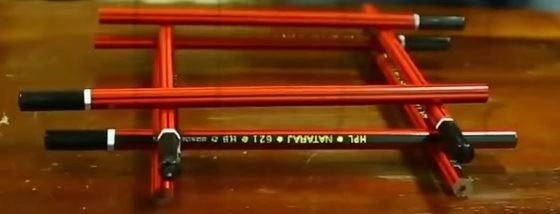There are a total of six pencils in this arrangement. The above arrangement contains three layers, and each layer has a fixed count of two pencils. The number of pencils in each layer never varies, but the number of layers you wish to build is entirely up to you.

Current Number of Layers = 4

Number of Pencils per Layer = 2

Total Number of Pencils = 2 x 4 = 8

What if you increase the number of layers to 10? What if you keep building up to a layer of 100? Can you sit and stack those many layers? Here, the answer is obviously NO. Instead, let’s try to calculate.

Number of Layers = 100

Number of Pencils per Layer = 2

Total Number of Pencils = 2 x 100 = 200

There is an obvious pattern here. A single level has 2 pencils, which is always constant, regardless of the number of levels built. So to get the total number of pencils, we have to multiply 2 (the number of pencils per level) with the number of levels built. For example, to construct 30 levels, you will need 2 multiplied 30 times which is 60 pencils.

According to the previous calculation, to make a building of ‘x’ number of levels, we will require 2 multiplied ‘x’ times, and thus the number of pencils equal to 2x. We just created algebraic expressions based on patterns. In this way, we can make several algebra patterns.

## Algebra as generalized Arithmetic Patterns

There are different types of algebraic patterns such as repeating patterns, growth patterns, number patterns, etc. All these patterns can be defined using different techniques. Let’s go through the algebra patterns using matchsticks given below.

## Algebra Matchstick Patterns

It is possible to make patterns with very basic things that we are using in our everyday life. Look at the following matchstick pattern of squares in the below figure. The squares are not separate. Two neighbouring squares have a common matchstick. Let’s observe the patterns and try to find the rule that gives the number of matchsticks.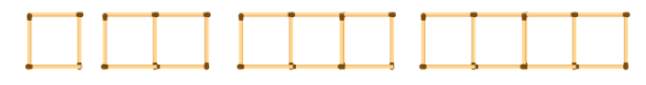In the above matchstick pattern, the number of matchsticks is 4, 7, 10 and 13, which is one more than the thrice of the number of squares in the pattern.

Therefore, this pattern can be defined using the algebraic expression 3x + 1, where x is the number of squares.

Now, let’s make the triangle pattern using matchsticks as shown in the below figure. Here, the triangles are connected with each other.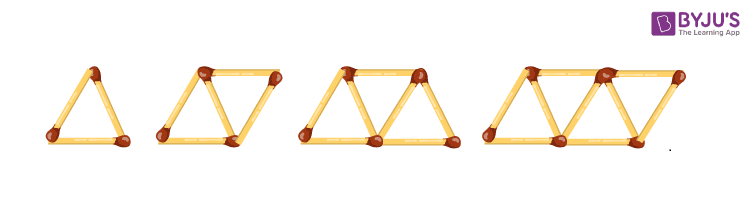In this matchstick pattern, the number of matchsticks is 3, 5, 7 and 9, which is one more than twice the number of triangles in the pattern. Therefore, the pattern is 2x + 1, where x is the number of triangles.

### How to do Number Patterns

Let’s look at another pattern. Say we have a triangle.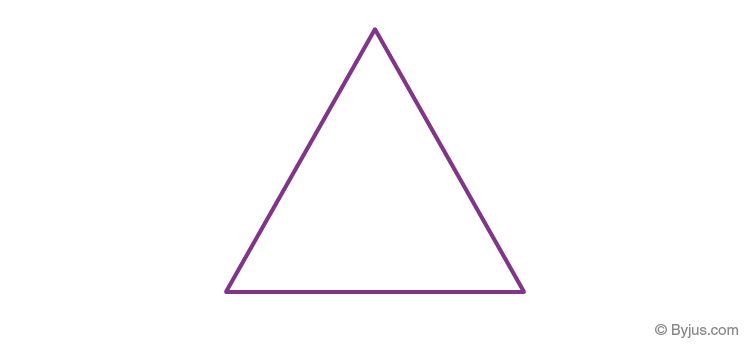Flip the triangle downwards and complete this image to form a complete triangle as given below: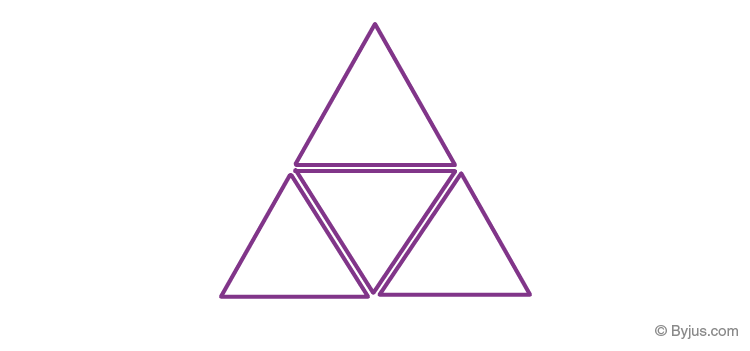The pattern is still a triangle, but the number of smaller triangles increases to 4. This bigger triangle now has two rows. What happens when we increase the number of rows and fill in the gaps with smaller triangles to complete that big triangle?

As we proceed to the 3rd row, how many smaller triangles do we have in total now? Now, this triangle has 9 smaller triangles.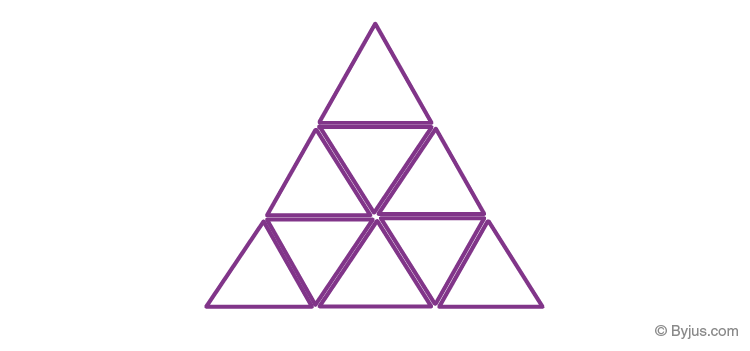What is the pattern here?

When,

r = 1, total no of triangles, ‘n’ = 1

When,

r = 2, n = 4

And when,

r = 3, n = 9

This can be summarised as:

 Number of rows 1 2 3 Number of smaller triangles 1 4 9

You can see that as the size of the triangle increases, the number of smaller triangles also increases. This means that n is just equal to r², which is 1², 2², 3² = 1, 4, 9…

So how do we represent this triangular pattern algebraically? r²! That’s it! We have just defined patterns algebraically. Also, the above pattern can be defined as the growth pattern in algebra.

We can write the sequence of odd numbers like 1, 3, 5, 7, 9……………………….. (2n – 1). If we substitute ‘n’ values in the expression beginning from one for odd numbers, i.e. 2n – 1, we can easily calculate the nth odd number. Substitute n= 11 to find the 11th odd number, and the result is 21, i.e. 11th odd number. Similarly, the 100th odd number is 199. Thus, the algebraic expression representing a set of odd numbers is 2n -1. It must be noted that n is a set of whole numbers in this case.

Similar to patterns in numbers, we can figure out the pattern in figures. For example, consider the following figures: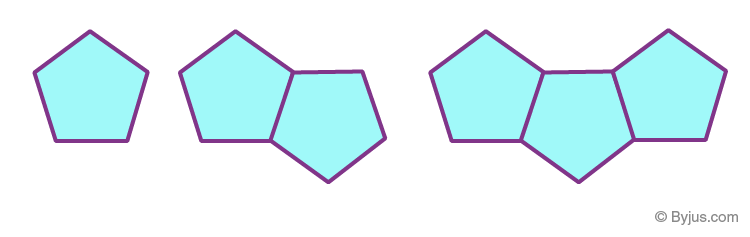In the figure given above, the first image is a pentagon with five sides. In the following figure, two pentagons are joined end to end, and the total number of sides is 9; in the next figure, the total number of sides is 13. It is observed that every other figure has 4 extra sides as compared to the previous one. Thus, the algebraic pattern that would define this sequence exactly is ‘4n + 1’, where n is any natural number. Therefore, if we substitute n = 3, we get the number of sides equal to 13. The 10th pattern in this sequence will have sides equal to 4 × 10 + 1, i.e. 41 sides.